Скачать презентацию COMP 171 Fall 2005 Analysis of Algorithms Review

d84b000a82528590d303d9400d2cf317.ppt

• Количество слайдов: 32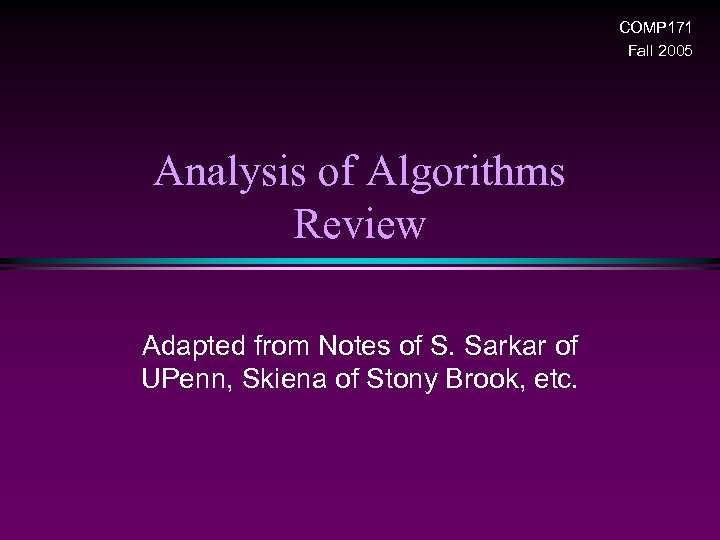COMP 171 Fall 2005 Analysis of Algorithms Review Adapted from Notes of S. Sarkar of UPenn, Skiena of Stony Brook, etc.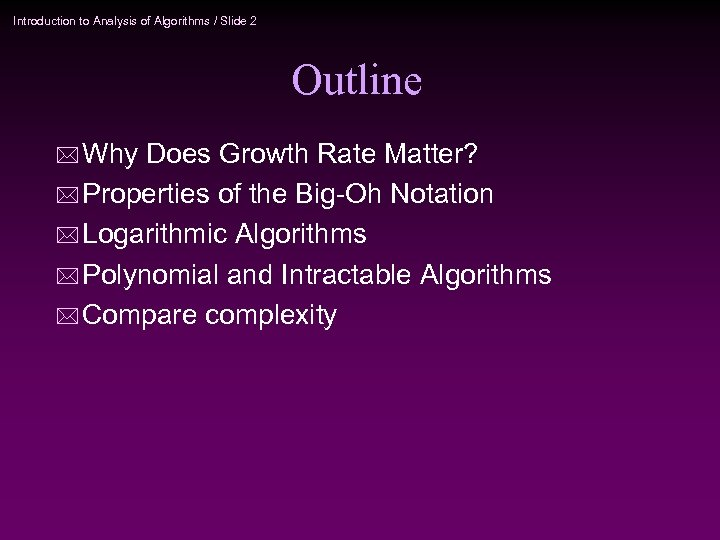Introduction to Analysis of Algorithms / Slide 2 Outline * Why Does Growth Rate Matter? * Properties of the Big-Oh Notation * Logarithmic Algorithms * Polynomial and Intractable Algorithms * Compare complexity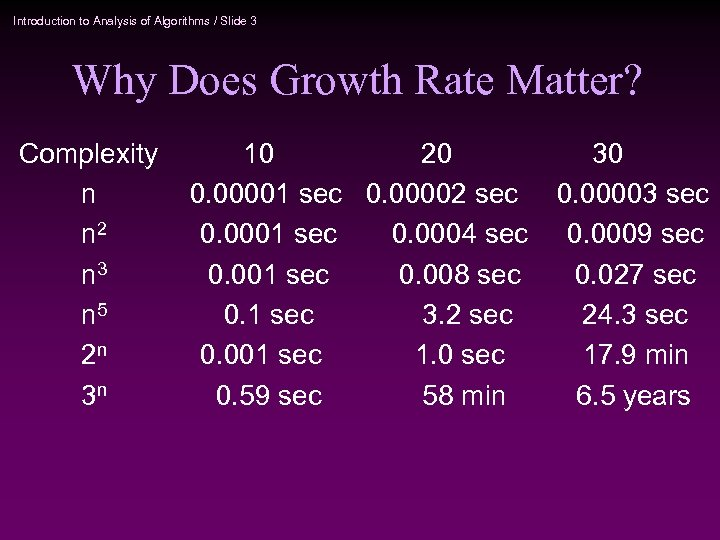Introduction to Analysis of Algorithms / Slide 3 Why Does Growth Rate Matter? Complexity n n 2 n 3 n 5 2 n 3 n 10 20 0. 00001 sec 0. 00002 sec 0. 0001 sec 0. 0004 sec 0. 001 sec 0. 008 sec 0. 1 sec 3. 2 sec 0. 001 sec 1. 0 sec 0. 59 sec 58 min 30 0. 00003 sec 0. 0009 sec 0. 027 sec 24. 3 sec 17. 9 min 6. 5 years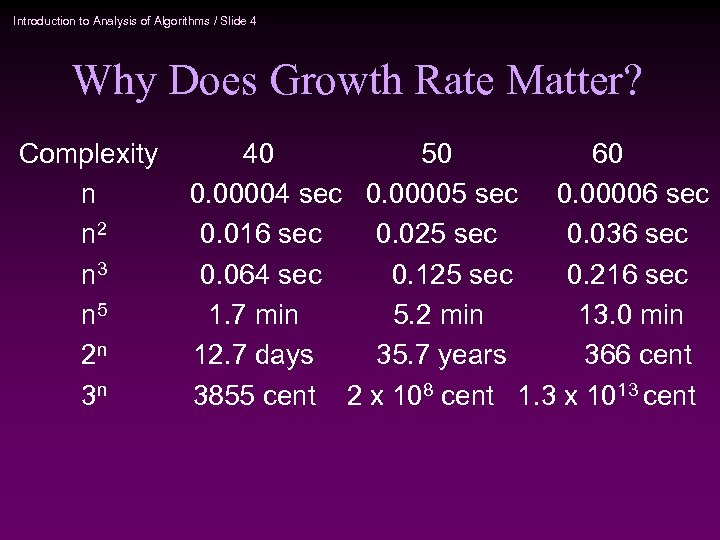Introduction to Analysis of Algorithms / Slide 4 Why Does Growth Rate Matter? Complexity n n 2 n 3 n 5 2 n 3 n 40 50 60 0. 00004 sec 0. 00005 sec 0. 00006 sec 0. 016 sec 0. 025 sec 0. 036 sec 0. 064 sec 0. 125 sec 0. 216 sec 1. 7 min 5. 2 min 13. 0 min 12. 7 days 35. 7 years 366 cent 3855 cent 2 x 108 cent 1. 3 x 1013 cent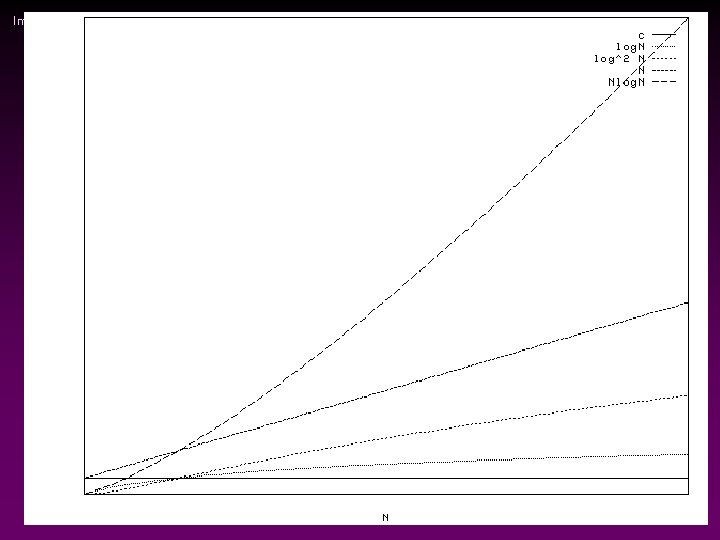Introduction to Analysis of Algorithms / Slide 5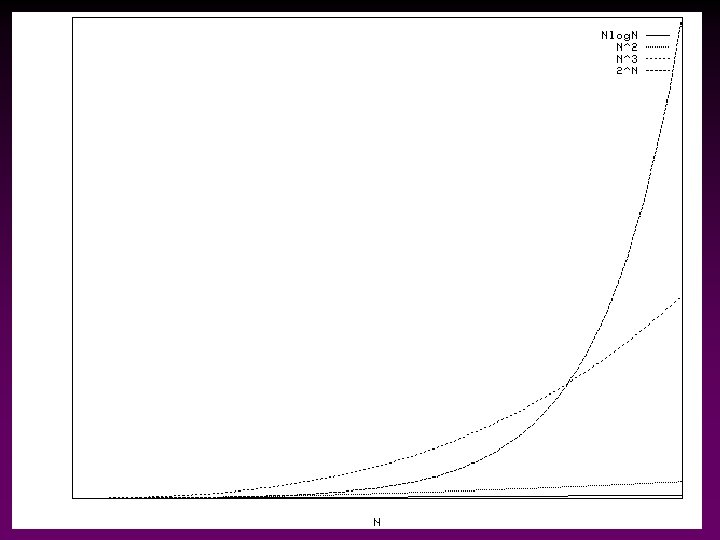Introduction to Analysis of Algorithms / Slide 6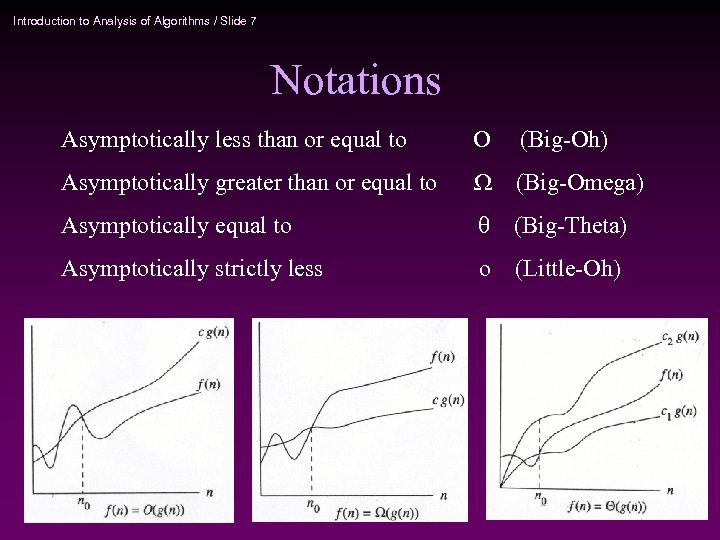Introduction to Analysis of Algorithms / Slide 7 Notations Asymptotically less than or equal to O (Big-Oh) Asymptotically greater than or equal to (Big-Omega) Asymptotically equal to (Big-Theta) Asymptotically strictly less o (Little-Oh)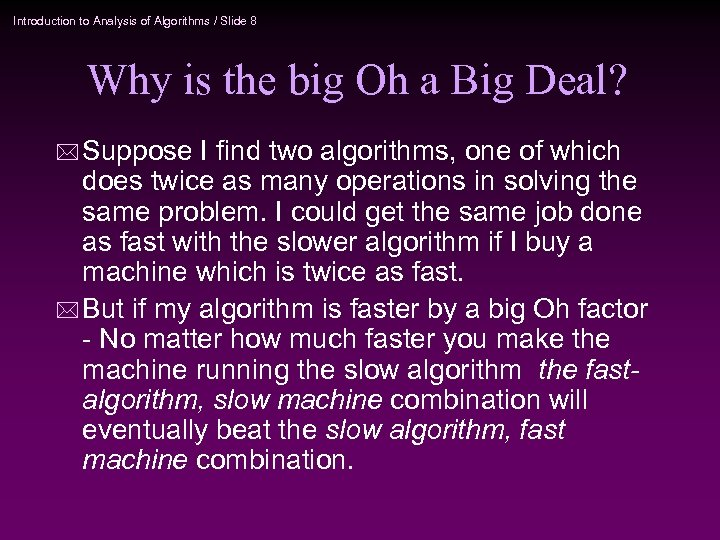Introduction to Analysis of Algorithms / Slide 8 Why is the big Oh a Big Deal? * Suppose I find two algorithms, one of which does twice as many operations in solving the same problem. I could get the same job done as fast with the slower algorithm if I buy a machine which is twice as fast. * But if my algorithm is faster by a big Oh factor - No matter how much faster you make the machine running the slow algorithm the fastalgorithm, slow machine combination will eventually beat the slow algorithm, fast machine combination.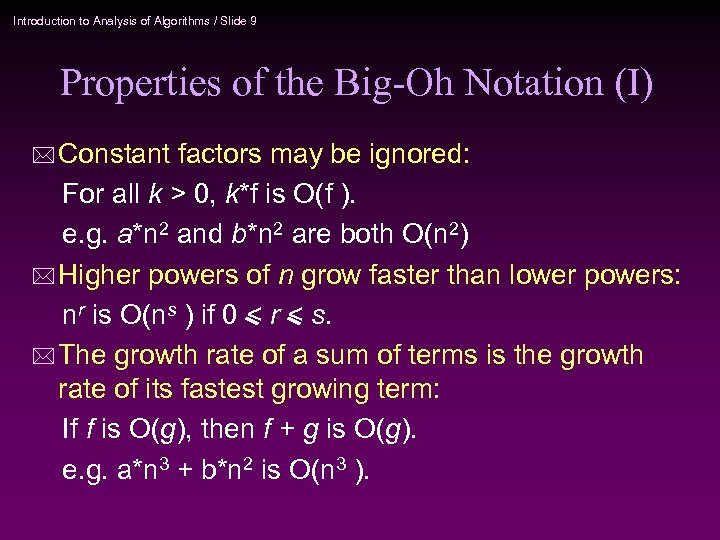Introduction to Analysis of Algorithms / Slide 9 Properties of the Big-Oh Notation (I) * Constant factors may be ignored: For all k > 0, k*f is O(f ). e. g. a*n 2 and b*n 2 are both O(n 2) * Higher powers of n grow faster than lower powers: nr is O(ns ) if 0 < r < s. * The growth rate of a sum of terms is the growth rate of its fastest growing term: If f is O(g), then f + g is O(g). e. g. a*n 3 + b*n 2 is O(n 3 ).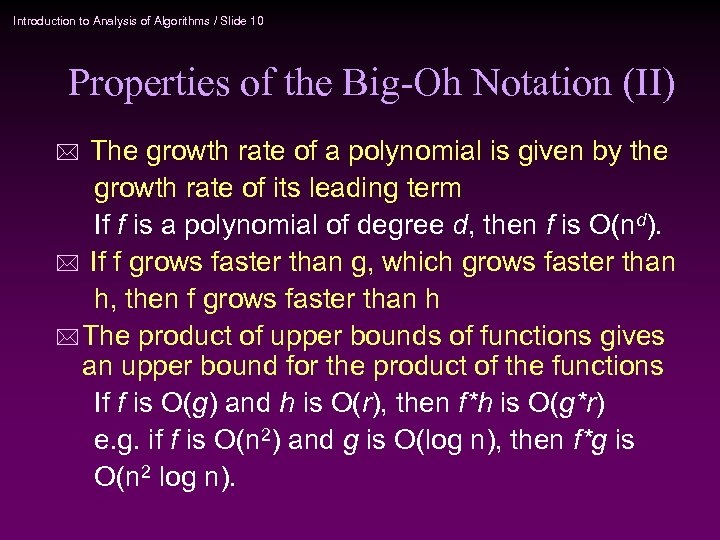Introduction to Analysis of Algorithms / Slide 10 Properties of the Big-Oh Notation (II) The growth rate of a polynomial is given by the growth rate of its leading term If f is a polynomial of degree d, then f is O(nd). * If f grows faster than g, which grows faster than h, then f grows faster than h * The product of upper bounds of functions gives an upper bound for the product of the functions If f is O(g) and h is O(r), then f*h is O(g*r) e. g. if f is O(n 2) and g is O(log n), then f*g is O(n 2 log n). *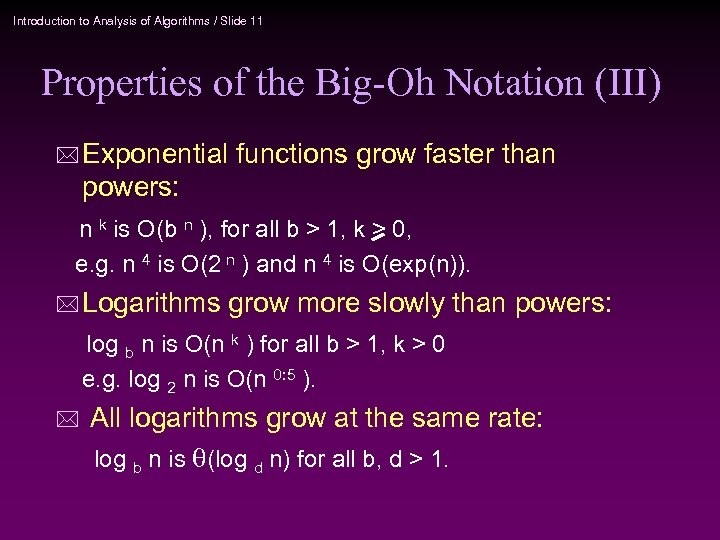Introduction to Analysis of Algorithms / Slide 11 Properties of the Big-Oh Notation (III) * Exponential functions grow faster than powers: n k is O(b n ), for all b > 1, k > 0, e. g. n 4 is O(2 n ) and n 4 is O(exp(n)). * Logarithms grow more slowly than powers: log b n is O(n k ) for all b > 1, k > 0 e. g. log 2 n is O(n 0: 5 ). * All logarithms grow at the same rate: log b n is (log d n) for all b, d > 1.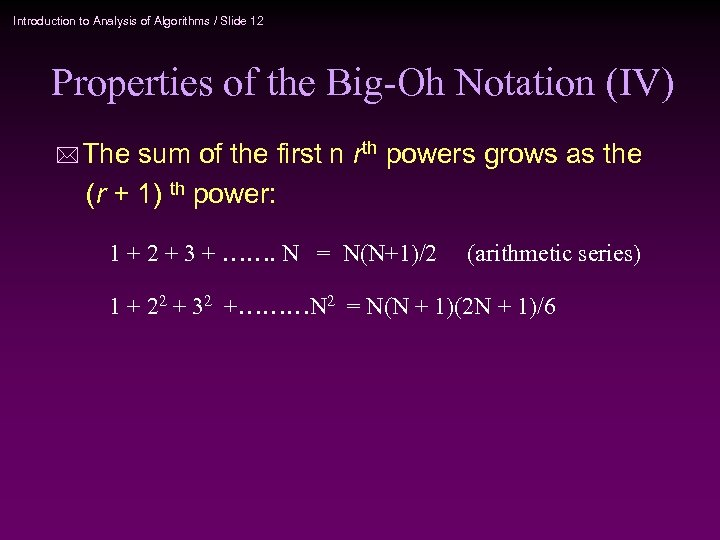Introduction to Analysis of Algorithms / Slide 12 Properties of the Big-Oh Notation (IV) * The sum of the first n rth powers grows as the (r + 1) th power: 1 + 2 + 3 + ……. N = N(N+1)/2 (arithmetic series) 1 + 22 + 32 +………N 2 = N(N + 1)(2 N + 1)/6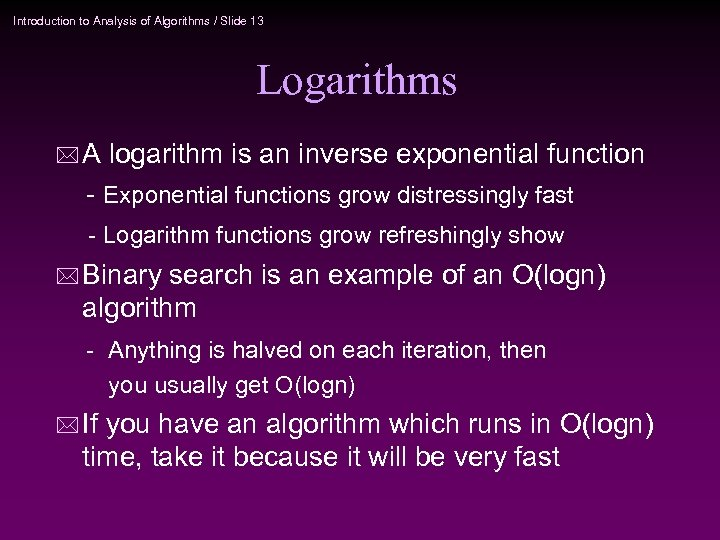Introduction to Analysis of Algorithms / Slide 13 Logarithms *A logarithm is an inverse exponential function - Exponential functions grow distressingly fast - Logarithm functions grow refreshingly show * Binary search is an example of an O(logn) algorithm - Anything is halved on each iteration, then you usually get O(logn) * If you have an algorithm which runs in O(logn) time, take it because it will be very fast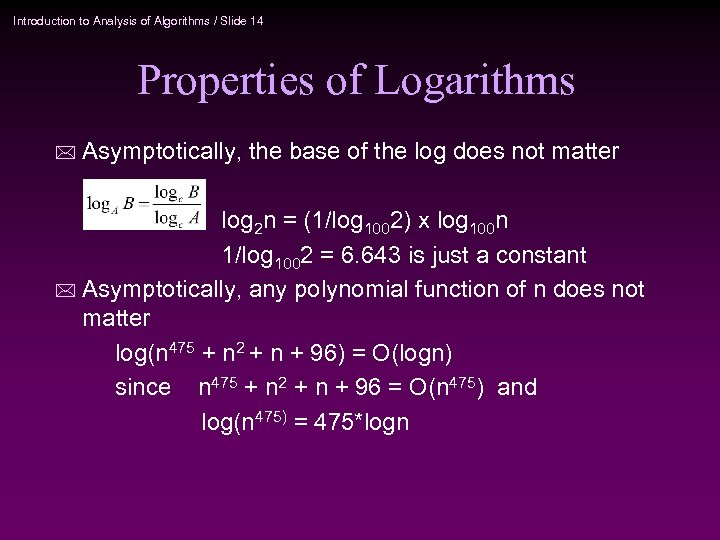Introduction to Analysis of Algorithms / Slide 14 Properties of Logarithms * Asymptotically, the base of the log does not matter log 2 n = (1/log 1002) x log 100 n 1/log 1002 = 6. 643 is just a constant * Asymptotically, any polynomial function of n does not matter log(n 475 + n 2 + n + 96) = O(logn) since n 475 + n 2 + n + 96 = O(n 475) and log(n 475) = 475*logn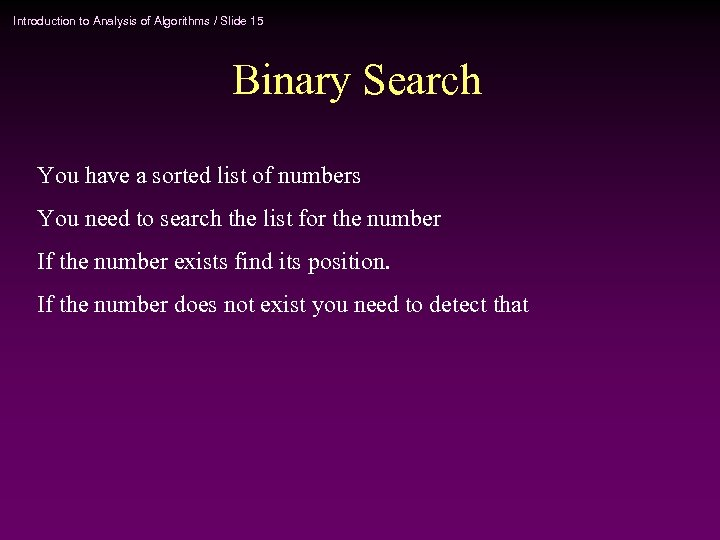Introduction to Analysis of Algorithms / Slide 15 Binary Search You have a sorted list of numbers You need to search the list for the number If the number exists find its position. If the number does not exist you need to detect that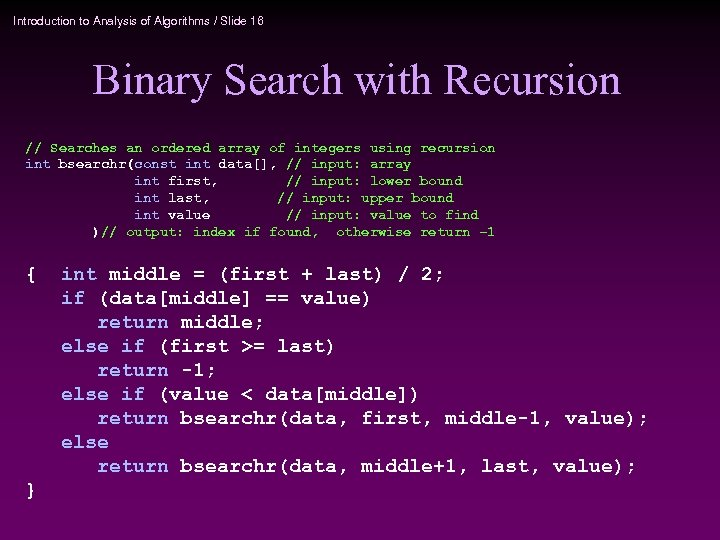Introduction to Analysis of Algorithms / Slide 16 Binary Search with Recursion // Searches an ordered array of integers using recursion int bsearchr(const int data[], // input: array int first, // input: lower bound int last, // input: upper bound int value // input: value to find )// output: index if found, otherwise return – 1 { } int middle = (first + last) / 2; if (data[middle] == value) return middle; else if (first >= last) return -1; else if (value < data[middle]) return bsearchr(data, first, middle-1, value); else return bsearchr(data, middle+1, last, value);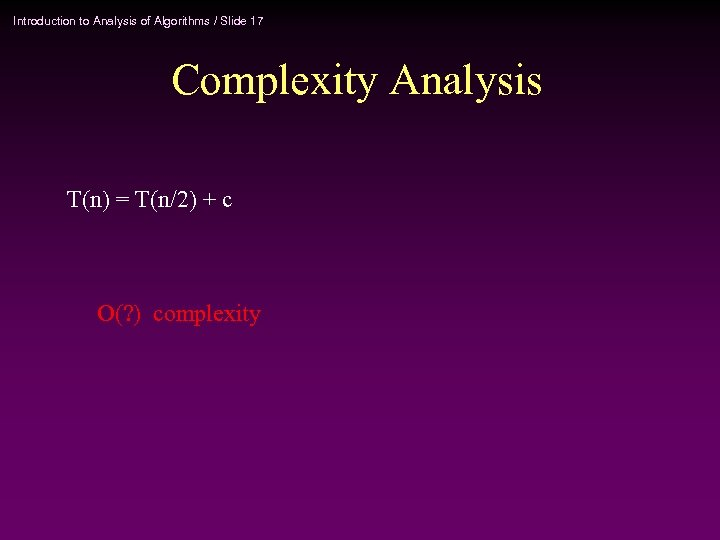Introduction to Analysis of Algorithms / Slide 17 Complexity Analysis T(n) = T(n/2) + c O(? ) complexity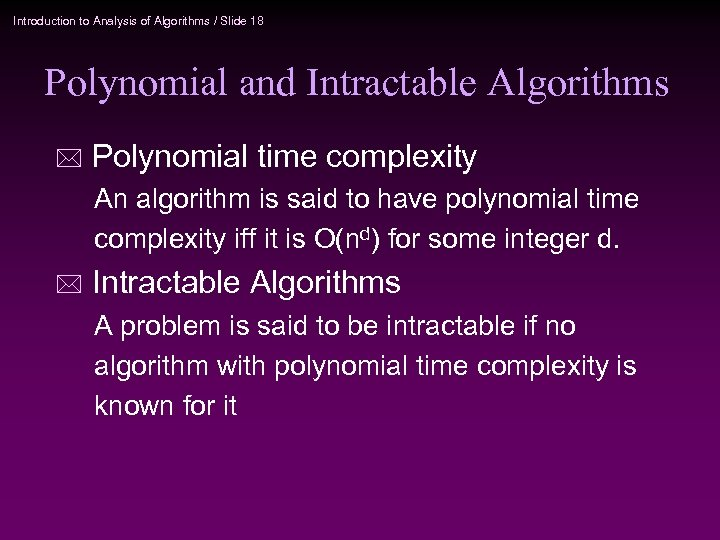Introduction to Analysis of Algorithms / Slide 18 Polynomial and Intractable Algorithms * Polynomial time complexity An algorithm is said to have polynomial time complexity iff it is O(nd) for some integer d. * Intractable Algorithms A problem is said to be intractable if no algorithm with polynomial time complexity is known for it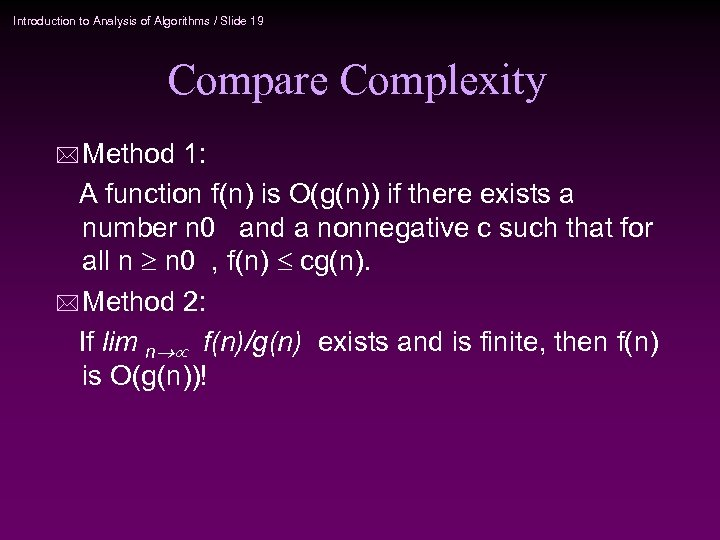Introduction to Analysis of Algorithms / Slide 19 Compare Complexity * Method 1: A function f(n) is O(g(n)) if there exists a number n 0 and a nonnegative c such that for all n n 0 , f(n) cg(n). * Method 2: If lim n f(n)/g(n) exists and is finite, then f(n) is O(g(n))!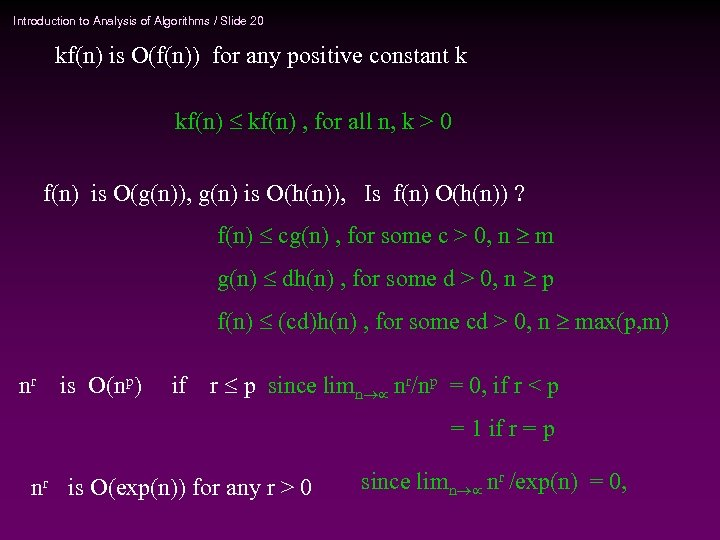Introduction to Analysis of Algorithms / Slide 20 kf(n) is O(f(n)) for any positive constant k kf(n) , for all n, k > 0 f(n) is O(g(n)), g(n) is O(h(n)), Is f(n) O(h(n)) ? f(n) cg(n) , for some c > 0, n m g(n) dh(n) , for some d > 0, n p f(n) (cd)h(n) , for some cd > 0, n max(p, m) nr is O(np) if r p since limn nr/np = 0, if r < p = 1 if r = p nr is O(exp(n)) for any r > 0 since limn nr /exp(n) = 0,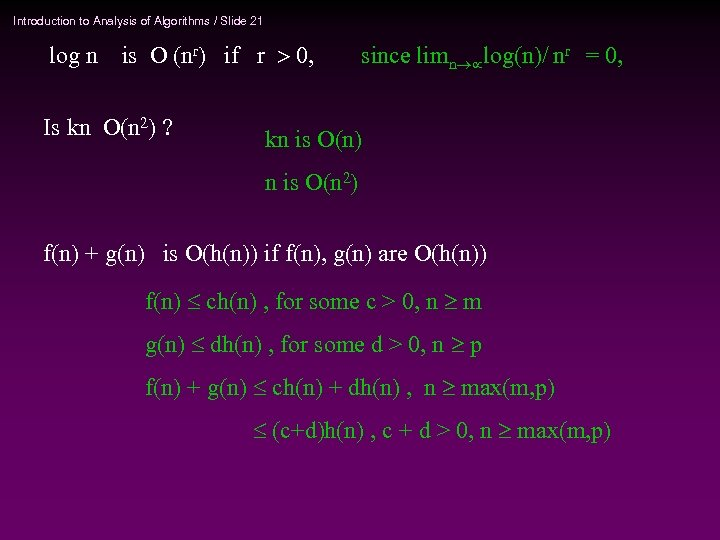Introduction to Analysis of Algorithms / Slide 21 log n is O (nr) if r 0, Is kn O(n 2) ? since limn log(n)/ nr = 0, kn is O(n) n is O(n 2) f(n) + g(n) is O(h(n)) if f(n), g(n) are O(h(n)) f(n) ch(n) , for some c > 0, n m g(n) dh(n) , for some d > 0, n p f(n) + g(n) ch(n) + dh(n) , n max(m, p) (c+d)h(n) , c + d > 0, n max(m, p)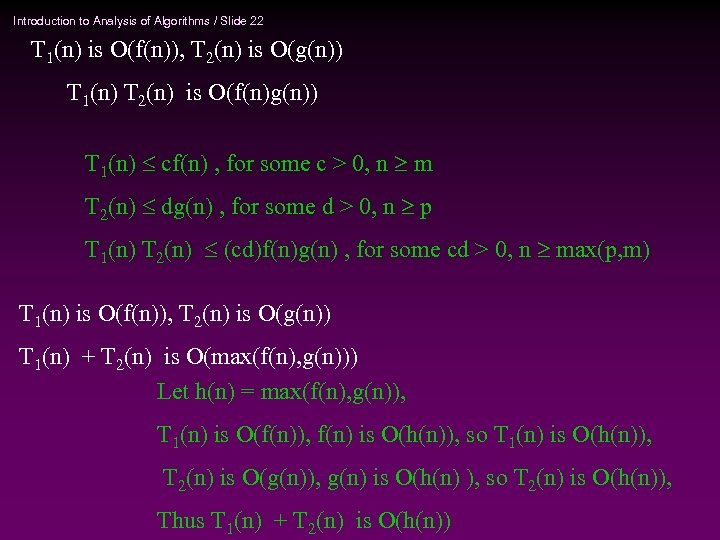Introduction to Analysis of Algorithms / Slide 22 T 1(n) is O(f(n)), T 2(n) is O(g(n)) T 1(n) T 2(n) is O(f(n)g(n)) T 1(n) cf(n) , for some c > 0, n m T 2(n) dg(n) , for some d > 0, n p T 1(n) T 2(n) (cd)f(n)g(n) , for some cd > 0, n max(p, m) T 1(n) is O(f(n)), T 2(n) is O(g(n)) T 1(n) + T 2(n) is O(max(f(n), g(n))) Let h(n) = max(f(n), g(n)), T 1(n) is O(f(n)), f(n) is O(h(n)), so T 1(n) is O(h(n)), T 2(n) is O(g(n)), g(n) is O(h(n) ), so T 2(n) is O(h(n)), Thus T 1(n) + T 2(n) is O(h(n))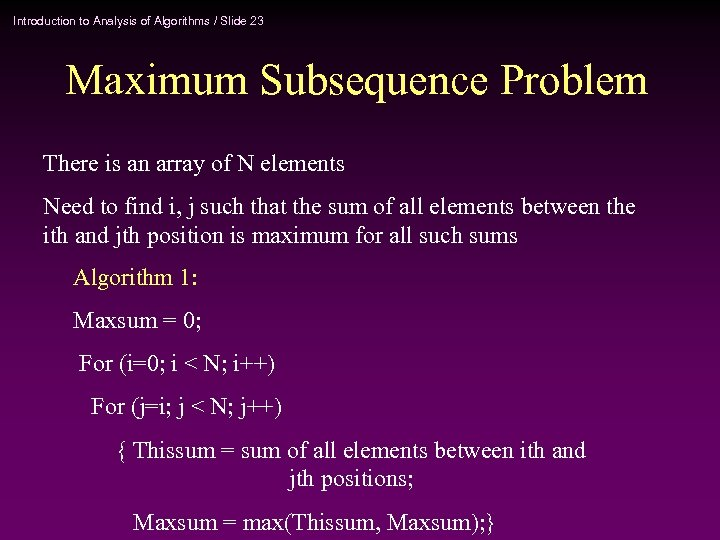Introduction to Analysis of Algorithms / Slide 23 Maximum Subsequence Problem There is an array of N elements Need to find i, j such that the sum of all elements between the ith and jth position is maximum for all such sums Algorithm 1: Maxsum = 0; For (i=0; i < N; i++) For (j=i; j < N; j++) { Thissum = sum of all elements between ith and jth positions; Maxsum = max(Thissum, Maxsum); }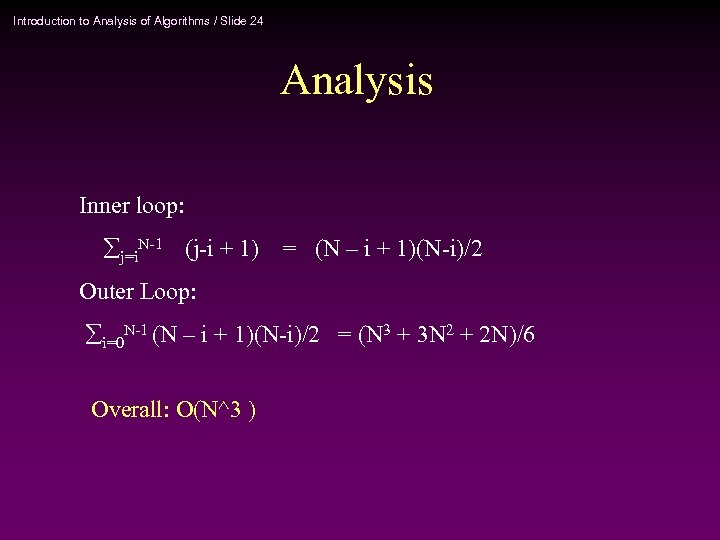Introduction to Analysis of Algorithms / Slide 24 Analysis Inner loop: j=i. N-1 (j-i + 1) = (N – i + 1)(N-i)/2 Outer Loop: i=0 N-1 (N – i + 1)(N-i)/2 = (N 3 + 3 N 2 + 2 N)/6 Overall: O(N^3 )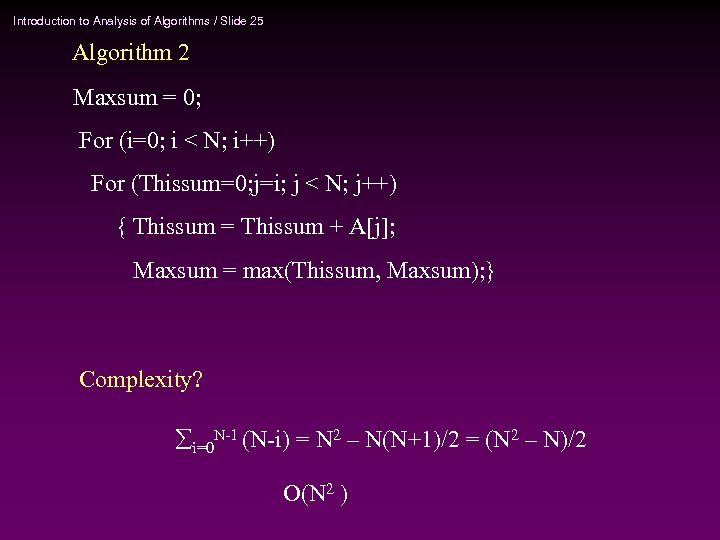Introduction to Analysis of Algorithms / Slide 25 Algorithm 2 Maxsum = 0; For (i=0; i < N; i++) For (Thissum=0; j=i; j < N; j++) { Thissum = Thissum + A[j]; Maxsum = max(Thissum, Maxsum); } Complexity? i=0 N-1 (N-i) = N 2 – N(N+1)/2 = (N 2 – N)/2 O(N 2 )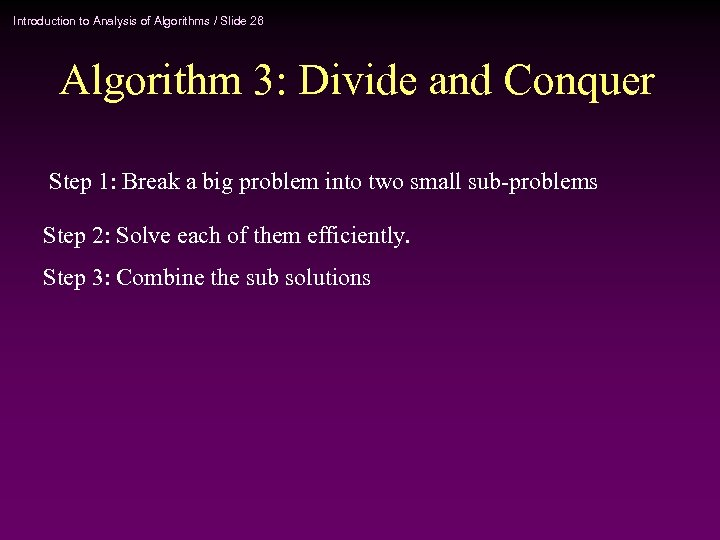Introduction to Analysis of Algorithms / Slide 26 Algorithm 3: Divide and Conquer Step 1: Break a big problem into two small sub-problems Step 2: Solve each of them efficiently. Step 3: Combine the sub solutions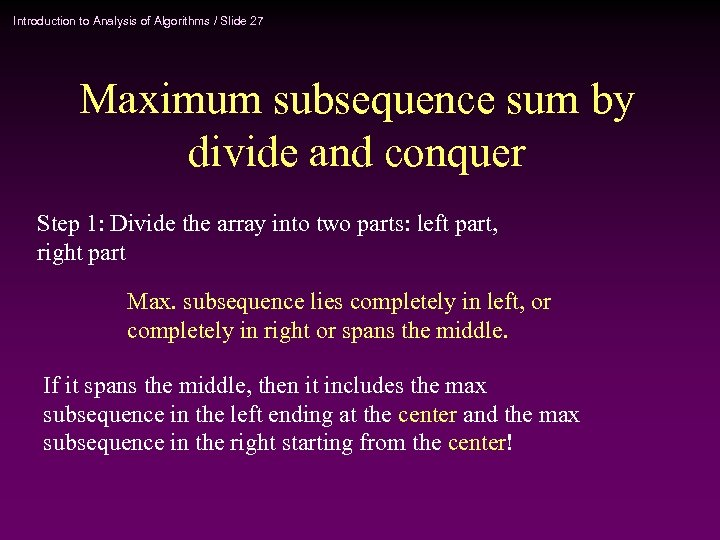Introduction to Analysis of Algorithms / Slide 27 Maximum subsequence sum by divide and conquer Step 1: Divide the array into two parts: left part, right part Max. subsequence lies completely in left, or completely in right or spans the middle. If it spans the middle, then it includes the max subsequence in the left ending at the center and the max subsequence in the right starting from the center!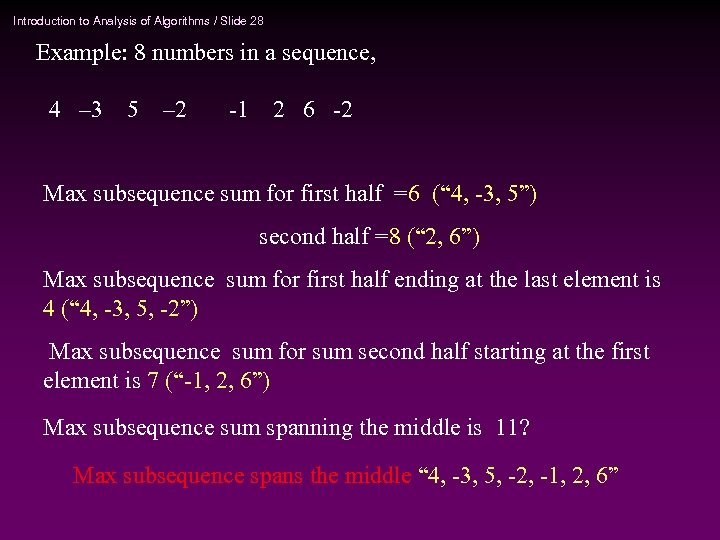Introduction to Analysis of Algorithms / Slide 28 Example: 8 numbers in a sequence, 4 – 3 5 – 2 -1 2 6 -2 Max subsequence sum for first half =6 (“ 4, -3, 5”) second half =8 (“ 2, 6”) Max subsequence sum for first half ending at the last element is 4 (“ 4, -3, 5, -2”) Max subsequence sum for sum second half starting at the first element is 7 (“-1, 2, 6”) Max subsequence sum spanning the middle is 11? Max subsequence spans the middle “ 4, -3, 5, -2, -1, 2, 6”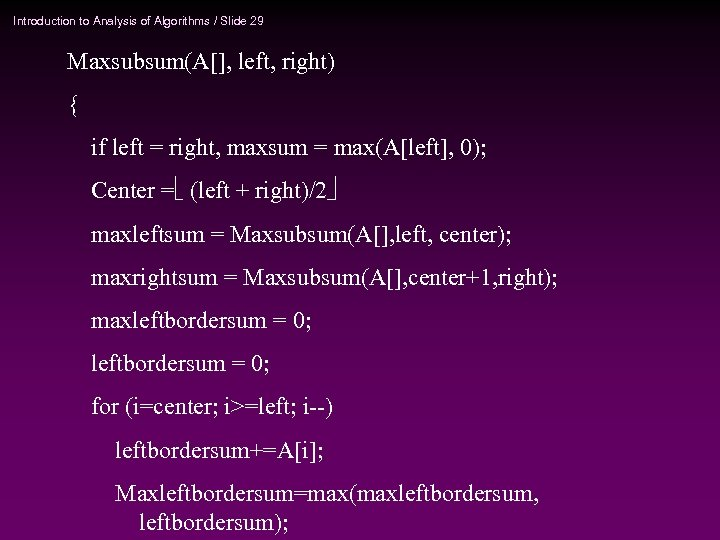Introduction to Analysis of Algorithms / Slide 29 Maxsubsum(A[], left, right) { if left = right, maxsum = max(A[left], 0); Center = (left + right)/2 maxleftsum = Maxsubsum(A[], left, center); maxrightsum = Maxsubsum(A[], center+1, right); maxleftbordersum = 0; for (i=center; i>=left; i--) leftbordersum+=A[i]; Maxleftbordersum=max(maxleftbordersum, leftbordersum);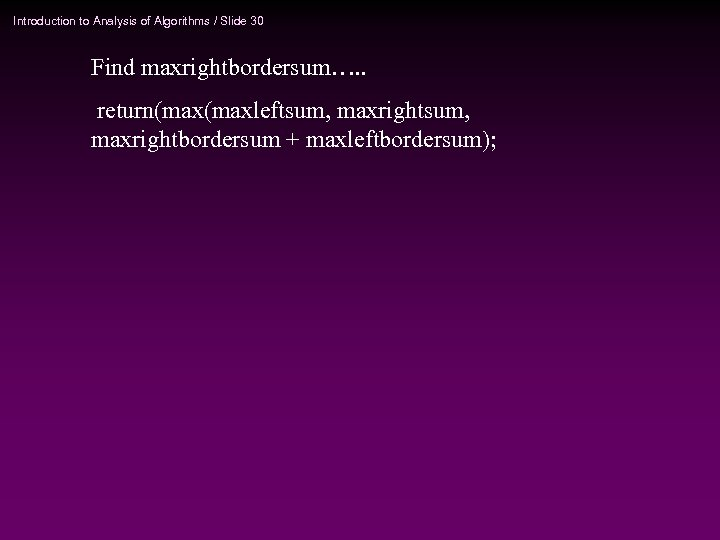Introduction to Analysis of Algorithms / Slide 30 Find maxrightbordersum…. . return(maxleftsum, maxrightbordersum + maxleftbordersum);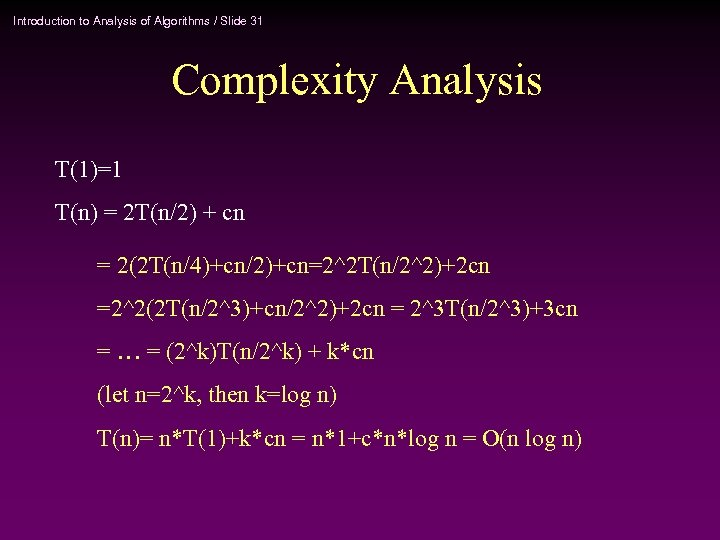Introduction to Analysis of Algorithms / Slide 31 Complexity Analysis T(1)=1 T(n) = 2 T(n/2) + cn = 2(2 T(n/4)+cn/2)+cn=2^2 T(n/2^2)+2 cn =2^2(2 T(n/2^3)+cn/2^2)+2 cn = 2^3 T(n/2^3)+3 cn = … = (2^k)T(n/2^k) + k*cn (let n=2^k, then k=log n) T(n)= n*T(1)+k*cn = n*1+c*n*log n = O(n log n)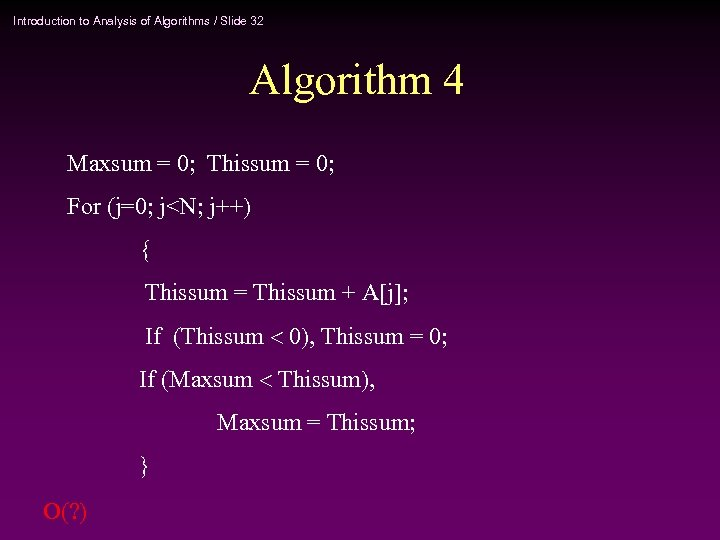Introduction to Analysis of Algorithms / Slide 32 Algorithm 4 Maxsum = 0; Thissum = 0; For (j=0; j